Verifying and#8216;Einsteinand#8217;s Timeand#8217; by Using the Equation and#8216;Time=Distance/Velocity | OMICS International
Journal of Physical Mathematics

# Verifying ‘Einstein’s Time’ by Using the Equation ‘Time=Distance/Velocity

Makanae M*

Independent Researcher, Representative Free Web College, Nishikasai, Edogawa-ku, Tokyo 134-0088, Japan

*Corresponding Author:
Makanae M
Independent Researcher
Representative Free Web College
Nishikasai, Edogawa-ku, Tokyo 134-0088, Japan
Tel: +81-(0)3-3878-9542
E-mail: [email protected]

Received Date: February 22, 2017; Accepted Date: March 03, 2017; Published Date: March 07, 2017

Citation: Makanae M (2017) Verifying ‘Einstein’s Time’ by Using the Equation ‘Time=Distance/Velocity. J Phys Math 8: 215. doi: 10.4172/2090-0902.1000215

Copyright: © 2017 Makanae M. This is an open-access article distributed under the terms of the Creative Commons Attribution License, which permits unrestricted use, distribution, and reproduction in any medium, provided the original author and source are credited.

Visit for more related articles at Journal of Physical Mathematics

#### Abstract

The statement ‘Every reference-body (co-ordinate system) has its own particular time’, which appears in Einstein’s book—‘Relativity: The Special and General Theory’, is widely accepted among physicists and even by the general public with the popular interpretation that a clock in a moving body and another clock at rest in the reference stationary body will indicate different values of time. However, upon examining the grounds for this perspective by using the equation ‘time=distance/velocity’ and using ‘the principle of the constancy of the velocity of light’, we find that the above sentence should be arranged as ‘Every reference body (coordinate system) has its own particular measurement of the time interval for the propagation of light and, also it has its own particular measurement of the interval of the light path that must be used in order to calculate its time interval. The numerical value of the ratio of these two intervals is 1:1 always.’ This implies that the pace of ticking of all clocks is identical. This fact contradicts the above popular interpretation.

#### Keywords

Numerical value; Einstein’s time; Lorentz factor

#### Introduction

In 1905, A. Einstein published the article titled ‘On the Electrodynamics of Moving Bodies’ , which is referred to as the Special Theory of Relativity (STR). In Section 1 of , he first defined ‘time’, which is obtained by observing the hands of a watch at the place where the watch and the observer (i.e., the user of the watch) are located. Then, he describes the method for confirming the synchronization of two clocks that are placed a certain distance apart by using the round trip of the ray of light between the two clocks. In Section 2 of , by considering the relationship between a moving system in which the round trip of the ray of light is performed and a reference stationary system in which an observer measures the time intervals of the round trip of the ray of light, Einstein implied that the progress of time in the moving system and the progress of time in the reference stationary system are different. Subsequently, in Section 3 of , he developed the expression,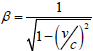, which was later termed as ‘Lorentz factor’, as a core theory of STR. This expression denotes the ratio between the value of ‘time’ or ‘length’ in the moving system and the value of ‘time’ or ‘length’ in the reference stationary system.

In 1916, A. Einstein published his book titled ‘Relativity: The Special and General Theory’ . In this book, as an explanation of STR, he introduced his thought experiment that consisted in the observation of the simultaneity of two lightning strikes. Based on this thought experiment, his conclusion regarding time was: ‘Every reference body (co-ordinate system) has its own particular time.’ (For convenience, hereafter, we refer to this Einstein’s perspective as ‘Einstein’s time’.)

Einstein’s time is widely accepted among physicists, and even among the general public, with the popular interpretation that a clock attached to a moving body and another clock at rest attached to a reference stationary body indicate different values of time. However, upon examining the grounds for this perspective by using the universal equation ‘time=distance/velocity’ and by incorporating ‘the principle of the constancy of the velocity of light’, we find that the above sentence should be arranged as ‘Every reference body (coordinate system) has its own particular measurement of the time interval for the propagation of light and, also, it has its own particular measurement of the interval of the light path that must be used in order to calculate its time interval. The numerical value of the ratio of these two intervals is always 1:1’. This indicates that the ticking pace of all clocks is identical. This fact contradicts the above popular interpretation.

In this study, we explain this issue with tangible reasons by using numerical values in a practical example. Then, we suggest a practical approach, which uses advanced technology of the 21st century, for verifying our conclusion, i.e., for verifying Einstein’s time.

#### Confirming the Definition of ‘Independent Time’

Before dwelling on Einstein’s time, we confirm the definition of the concept of ‘independent time’ (as we call it) that was provided in Section 1 of , as this concept is the starting point for studying Einstein’s perspective regarding ‘time’.

In the first half of Section 1 of , after emphasizing that the concept of time is important in the study of physics, Einstein considered ‘time’ at a place where a watch and an observer (i.e., the user of the watch) are located; then he states ‘the definition of “time” by substituting “the position of the small hand of my watch” for “time”’, hereafter referred to as Sentence 1.

We can consider sundials, hourglasses, modern digital clocks, or any other instrument that can be used to measure time, instead of the ‘hand’ of the watch that appears in Sentence 1. Therefore, we may replace Sentence 1 with ‘the definition of “time” by substituting “the indicator of my/a watch” for “time”’, which may be referred to as Sentence 2.

Usually, once a certain term has been replaced by another term, we can put the former term aside. In Sentence 1, ‘time’ (as the last word in the sentence) is replaced by ‘the indicator of a watch’. Therefore, we can arrange Sentence 2 as ‘the definition of “time” is “the indicator of watch (or clock)”’ by removing the phrase ‘for time’. This sentence is ideal from the viewpoint of formal logic, because it is meaningless to define the concept of time by using the phrase ‘for time’ in this case. Hereafter, we treat this sentence as a definition of the concept of ‘independent time’, for the reason that this concept is established at the place where a watch and an observer are located. We can say that this Einstein’s concept of independent time is correct, since time measurement can be carried out precisely and scientifically only by watching the indicator of a clock.

With the premise that Einstein’s definition of independent time is correct, we can state that the progress of (independent) time can only be known through a clock that ticks at a steady pace, unless the clock is out of order. Thus, we assume that the pace of time progress can be confirmed by the ticking pace of a clock.

#### Confirming the Background of the Primary Perspective of Einstein's Time

After explaining ‘independent time’, in Section 1 of , Einstein describes a method for the synchronization of two clocks in the same coordinate system by using the round trip of a ray of light between the two clocks as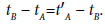(1)

This equation is established based on the following assumptions.

• A clock is located at point A, and another clock is located at point B.

• tA is the point of time when the ray is emitted from the light source located at A.

• tB is the point of time when the ray is reflected by a mirror placed at B.

• t′A is the point of time when the ray returns to A.

• The left hand side of (1) represents the time required for the ray to ‘Go’ (from A to B)

• The right hand side represents the time required for the ray to ‘Return’ (from B to A).

With the assumption that the velocity of light is constant in vacuum, the right-hand and left-hand sides of (1) are equal. Thus, we can conclude that the synchronization of the two clocks is satisfied. By assuming that the distance between points A and B (viewed within the system to which both the points belong) is 1l, the ray of light moves 1l in 1 s. Thus, we can express (1) as 1 s - 0 s=2 s - 1 s, then obtaining 1 s=1 s.

In Section 2 of , based on ‘the principle of relativity’, ‘the principle of the constancy of the velocity of light’, and the above method for confirming the synchronisation of the two clocks, Einstein considered the relationships between a moving system and a reference stationary system with the concepts of ‘time’ and ‘length’, using the universal equation ‘time=distance/velocity’. He then proved the following set of equations,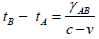and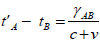(2)

The premises implied or described by Eq. (2) are the following:

1. Two coordinate systems: the moving system refers to a moving rigid rod, and the stationary system is described as the reference frame.

2. The rigid rod travels with a uniform velocity, undergoing parallel translation with respect to the stationary system along the positive direction of the x-axis.

3. A is the point at the beginning of the rod, closest to the origin of the x-axis, and B is the point at the end of the rod, at a distance l from A.

4. A light source is placed at A, and a mirror is placed at B to reflect the light in the opposite direction.

5. A clock is placed at each of the points A and B.

6. The round trip of a ray of light between A and B is governed by Eq. (1) in the moving system.

7. The velocity of the moving rigid rod is v, and c is the velocity of light.

8. The point in time at which the light is emitted by the light source is tA.

9. The point in time at which the light is reflected by the mirror is tB.

10. The point in time at which the light returns to A is t′A.

11. ‘γAB denotes the length of the moving rigid rod— measured in the stationary system’.

12. The quantity c−v or c+v is the relative velocity between the front of the ray and A viewed from the stationary reference system.

Conditions 4, 5, 6, 8, 9, and 10 are the same conditions expressed by Eq. (1).

When an observer at rest in the reference stationary system observes this round trip of the ray of light, Eq. (2) holds. The left side of Eq. (2) corresponds to the Go interval (A to B), and the right side corresponds to the Return interval (B to A). Unlike Eq. (1), the structure of the equations representing Go and Return is different in Eq. (2). Based on Eq. (2), Einstein concluded Section 2 of  with the following statement.

Observers moving with the moving rod would thus find that the two clocks were not synchronous, while observers in the stationary system would declare the clocks to be synchronous. So we see that we cannot attach any absolute signification to the concept of simultaneity, but that two events which, viewed from a system of co-ordinates, are simultaneous, can no longer be looked upon as simultaneous events when envisaged from a system which is in motion relatively to that system’. We can say that this sentence indirectly implies Einstein’s time. In other words, this sentence can be defined as a primary perspective of the Einstein’s time. Thus, for brevity, we refer to this sentence as the ‘primary perspective of Einstein’s time’.

#### Calculating the Time Intervals of the Trips of the Light Ray

First, let us calculate the time intervals in the round trip of the ray between two clocks by using numerical values in a practical example, where the velocity of the moving system is half of the velocity of light (i.e., 0.5c), and the distance between the two clocks placed at A and B is 1l viewed within the moving system. The ray of light travels 1l in 1 s. Hereafter, the clock placed at A is described as ‘Clock A’ and the clock placed at B is described as ‘Clock B’.

Whereas the moving system moves along the x-axis of the reference stationary system, the system appears to be stationary when viewed in the moving system, since it moves with uniform velocity. Therefore, in the moving system, in which the round trip of the ray between Clock A and Clock B is performed, we can calculate the time interval for Go and Return by using Eq. (1), namely tB − tA=t′A − tB. Thus, we obtain 1 s in both intervals. In other words, 1 s=1 s in the moving system.

On the other hand, if observing the Go interval of the round trip of the ray from the reference stationary system, when 1l +vt=ct, the mirror reflects the front of the ray under ‘the principle of the constancy of the velocity of light’. We can convert 1l+ vt=ct to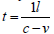.The time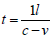corresponds to the time interval between tA and tB. Thus, we can express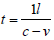as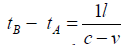(3)

Inserting the numerical value of v (i.e., 0.5c) in the denominator of the right-hand side, we obtain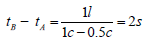Likewise, in the Return interval, if we assume for the time being that the point of time when the mirror reflects the front of the ray is 0 s, where 1l − vt=ct, the front of the ray returns to Clock A (viewed from the reference stationary system). We can convert 1l-vt=ct to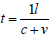. The time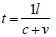corresponds to the time interval from tB to t'A. Thus, we can express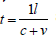as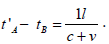(4)

Inserting the numerical value of v (i.e., 0.5c) in the denominator of the right-hand side, we obtain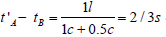. . From the above, we conclude that the time intervals for the Go and Return trips measured by the observer at rest in the reference stationary system are different, and are in the ratio 2 s:2/3 s, whereas, the proportion is 1 s:1 s when measured by the observer at rest in the moving system.

The above situation does not consider the Lorentz factor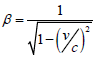, which is described in Section 3 of  as a core theory of STR. The denominator of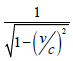denotes the value of ‘time’ or ‘length’ in a moving system, whereas the corresponding value in the reference stationary system is unity. Therefore, if the Lorentz factor is employed and the value of the velocity of the moving system (i.e., v) is 0.5c, the distance between A (where Clock A and the light source are placed) and B (where Clock B and the mirror are placed) is 1l viewed within the moving system, and the ray moves 1l in 1 s, then the value of the time interval in the moving system becomes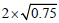s in the Go trip and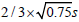in the Return trip. By inserting the numerical value of v (i.e., 0.5c) in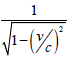, it becomes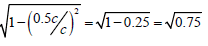, and the time interval for the light ray to travel as measured by the observer at rest in the reference stationary system equals 2 s in the Go trip and 2/3 s in the Return trip, as previously obtained.

From the above, we can infer that the time intervals of the same round trip of the ray are different in the reference stationary system and in the moving system when the Lorentz factor is employed. It seems that the results of the above calculation prove the correctness of Einstein’s time, namely ‘Every reference-body (co-ordinate system) has its own particular time’.

#### Considering ‘Time’ and ‘Distance’ in the Expression ‘Time=Distance’

In the previous section of our study, we focused on the time interval for the propagation of a ray of light. Now, we consider the above time intervals by including the ‘distance’ of the trips of the ray, i.e., the intervals of the light path between A (where Clock A and the light source are placed) and B (where Clock B and the mirror are placed).

By employing ‘the principle of the constancy of the velocity of light’, the velocity of the ray of light in the universal equation ‘time=distance/ velocity’ can be described as unity. Thus, we can treat ‘time’ and ‘distance’ as equivalent; we will write ‘time=distance’, for convenience. With the above assumption that time=distance and not employing the Lorenz factor, the relationship between the time interval for the propagation of the ray of light (i.e., ‘time’) and the interval for the path of the light ray (i.e., ‘distance’) can be expressed as 2 s=2l in the Go trip and 2/3 s=2/3l in the Return trip, when observing the round trip of the ray from the reference stationary system. On the other hand, the relationship is 1 s=1l in both the Go and Return trips when observing in the moving system.

If the Lorenz factor is employed and with the assumption that the time interval for the propagation of the light ray in the reference stationary system is 2 s and the interval for the light path of the ray is 2l in the Go trip, 2/3 s and 2/3l in the Return trip, we can describe time=distance as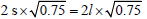in the Go trip, and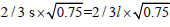in the Return trip, in the moving system.

From the above, we find that the interval for the path of the light ray per second is 1l in every situation, whether the Lorentz factor is employed or not. This means that the ratio of the numerical value of the time interval for the propagation of the ray of light to the interval of the path of the light ray is 1:1, which also implies that unless the clocks are out of order, the ticking pace of the clocks is always identical. In other words, all clocks are synchronous, even if each time interval for the propagation of the light ray has its own particular value.

#### Confirming the Transformation Method for the Case When the Lorentz Factor is not Employed

In the above statement, we considered two cases, one that employs the Lorentz factor and another that does not employ it. The Lorentz factor denotes the ratio of the value of ‘time’ (or ‘length’) in the reference stationary system to the value of ‘time’ (or ‘length’) in the moving system. Thus, the transformation method provides the ratio as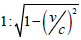. We treat below the case when the Lorentz factor is not employed.

We previously confirmed that the moving system has uniform velocity. Thus, this system is stationary when viewed within the system itself, and thus the ray of light moves 1l in 1 s in the Go and Return trips, so the interval of the light path between Clock A and Clock B viewed within the moving system is 1l. Therefore, t=1l/c. This equation corresponds to the form of the universal equation ‘time=distance/ velocity’.

On the other hand, as measured by the observer at rest in the reference stationary system, the time interval for the Go trip of the light ray is 2 s. This value is obtained by using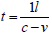, because, when 1l +vt=ct, the mirror reflects the front of the ray viewed from the reference stationary system. Therefore, with the equation time=distance/ velocity, we can express the ratio of the ‘time’ (interval) in the reference stationary system to the ‘time’ (interval) in the moving system as ‘1l/ (c-v):1l/c’. We can reduce ‘1l/(c-v):1l/c’ to ‘c:c - v’.

The Return interval of the round trip of the ray refers to the opposite of the Go interval. Thus, we can denote the above ratio as ‘c:c+v’. For confirming that this ratio for Return is correct, we can insert the numerical value of the practical example for v (i.e., 0.5c). We then obtain the numerical ratio as 1:1.5. This ratio matches the ratio 2/3 s:1 s that we previously obtained.

From the above, in the case when the Lorentz factor is not employed, the transformation theory gives the ratio for the round of trip of the ray as ‘c:c-v’ for the Go trip and ‘c:c+v’ for the Return trip.

#### Difference between Equations (2), (3), and (4)

Here, we notice that the structure of the set of equations (2) provided in Section 2 of , namely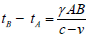and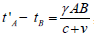, resembles the structure of equations (3) and (4), namely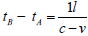and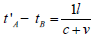, except that the expressions in the numerators of the right-hand side are different: γAB in (2), and 1l in (3) and (4), where γAB is ‘the length of the moving rigid rod—measured in the stationary system’. From the above, it seems that the correct numerical value of γAB is 1l.

However, Eq. (2) is established based on the concept of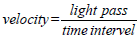, which can be arranged to time=distance/ velocity, described in the first half of Section 2 in . On the other hand, 1l in Eqs. (3) and (4) is the result of transposing from 1l+vt=ct or 1l − vt=ct, which is the time corresponding to the light propagation between Clock A and Clock B, as viewed from the reference stationary system. In other words, the grounds for γAB in Eq. (2) and 1l in Eqs. (3), and (4) are different, whereas the structures of Eq. (2) and Eqs. (3) and (4) resemble each other. Therefore, we cannot conclude that γAB in Eq. (2) equals 1l is correct. In fact, in Section 2 of , Einstein implies that the value of γAB differs from 1l. Therefore, this issue, where γAB of Eq. (2) and 1l in Eqs. (3) and (4) have different meanings in similar equations, does not affect our study in this paper, even if the tangible value of γAB is unknown. At any rate, in our study the main focus is not on Einstein’s ‘distance’ or ‘length of a rigid rod’, but on ‘time’. Therefore, we set aside the issue of γAB and concentrate on ‘time’ instead.

#### Confirming the Background of Einstein’s Time in 

From the above considerations, we can conclude that the primary perspective of Einstein’s time provided in  cannot be used as the ground of Einstein’s time provided in . Therefore, we now focus on Einstein’s time itself.

First, we confirm the background of Einstein’s time, namely ‘Every reference-body (co-ordinate system) has its own particular time’. Here, between Sections 1 and 17, Einstein explained regarding the background of STR and STR itself. From Section 18 to 29, he provided his perspective regarding the General Theory of Relativity. From Section 30 to Section 32, he introduced his perspective of our universe.

In Section 8 of, titled ‘On the Idea of Time in Physics,’ Einstein assumed a situation in which two lightning strikes occur simultaneously when a train runs along a straight railway embankment as a thought experiment. He then introduces a method to recognize the simultaneity of the two lightning strikes from the midpoint between Points A and B, where the lightning strikes the ground. Einstein described this method as follows:

By measuring along the rails, the connecting line AB should be measured up and an observer placed at the midpoint M of the distance AB. This observer should be supplied with an arrangement (e.g. two mirrors inclined at 90°) which allows him visually to observe both places A and B at the same time. If the observer perceives the two flashes of lightning at the same time, then they are simultaneous.’

Then, in Section 9, ‘The Relativity of Simultaneity,’ he explained the following:

When we say that the lightning strokes A and B are simultaneous with respect to the embankment, we mean: the rays of light emitted at the places A and B, where the lightning occurs, meet each other at the mid-point M of the length A → B of the embankment. However, the events A and B also correspond to positions A and B on the train. Let M' be the mid-point of the distance A → B on the travelling train. Just when the flashes 1 of lightning occur, this point M' naturally coincides with the point M, but it moves towards the right in the diagram with the velocity v of the train. If an observer sitting in the position M’ in the train did not possess this velocity, then he would remain permanently at M, and the light rays emitted by the flashes of lightning A and B would reach him simultaneously, i.e. they would meet just where he is situated. Now in reality (considered with reference to the railway embankment) he is hastening towards the beam of light coming from B, whilst he is riding on ahead of the beam of light coming from A. Hence the observer will see the beam of light emitted from B earlier than he will see that emitted from A. Observers who take the railway train as their reference-body must therefore come to the conclusion that the lightning flash B took place earlier than the lightning flash A.’

The above explanation of the thought experiment is correct and very clear, and is easily intelligible to the general public. Immediately after that explanation, Einstein stated the following conclusion of the above thought experiment, which includes Einstein’s time.

We thus arrive at the important result: Events which are simultaneous with reference to the embankment are not simultaneous with respect to the train, and vice versa (relativity of simultaneity). Every reference-body (co-ordinate system) has its own particular time; unless we are told the reference-body to which the statement of time refers, there is no meaning in a statement of the time of an event.’

#### Examining the Tangible Timings of the Flashes Reaching M or M′

Here, let us examine the tangible timings of the two flashes reaching M or M′ by using numerical values in a practical example.

First, we assume the following conditions, along with the conditions that A, B, and M are the stationary points on the embankment and point M′ moves with the train.

• The value of the distance from A or B to M is 1l (i.e., from A to B is 2l).

• The value of the distance from A or B to M′ is 1l, at 0 s.

• The flash of light moves 1l in 1s on the embankment and also in the train, satisfying ‘the principle of constancy of the velocity of light’.

• The velocity of the train, which is expressed as v, equals half the speed of light, i.e. 0.5c.

• The lightning strikes A and B simultaneously at 0 s.

• We denote the mirror placed at A as ‘Mirror A’, and that placed at B as ‘Mirror B’.

• We denote the flash reflected by Mirror A as ‘Flash A’, and that reflected by Mirror B as ‘Flash B’.

The following results are then obtained.

On the embankment:

• Flash A reaches M at 1 s.

• The interval of the light path of Flash A from Mirror A to M is 1l.

• Flash B reaches M at 1 s.

• The interval of the light path of Flash B from Mirror B to M is 1l. On the train:

• Flash A reaches M′ at 2 s.

• The interval of the light path of Flash A from Mirror A to M′ is 2l.

• Flash B reaches M′ at 2/3 s.

• The interval of the light path of Flash B from Mirror B to M′ is 2/3l.

From the above, we can confirm that the observer placed at M (i.e., on the embankment) recognizes both flashes at the same time, in contrast to the observer placed at M′ (i.e., on the train) who recognizes Flash B before Flash A. This result matches Einstein’s explanation in Section 9 of  ‘Observers who take the railway train as their referencebody must therefore come to the conclusion that the lightning flash B took place earlier than the lightning flash A.’

#### Confirming the Pace of Time Progress

Now, let us describe the form ‘time=distance/velocity’ by using the numerical values obtained in Section 2 of our study. We note that ‘interval of the light path of the flash’ corresponds to ‘distance’ in ‘time=distance / velocity’.

• For the interval of the light path of Flash A from Mirror A to M; 1 s=1l/c.

• For the interval of the light path of Flash B from Mirror B to M; 1 s=1l/c.

• For the interval of the light path of Flash A from Mirror A to M′; 2 s=2l/c.

• For the interval of the light path of Flash B from Mirror B to M′; 2/3 s=2/3l/c.

In ‘the principle of the constancy of the velocity of light’, we can describe c as unity. Thus, the above expressions become:

• For the interval of the light path of Flash A from Mirror A to M; 1 s=1l.

• For the interval of the light path of Flash B from Mirror B to M; 1 s=1l.

• For the interval of the light path of Flash A from Mirror A to M′; 2 s=2 l.

• For the interval of the light path of Flash B from Mirror B to M′; 2/3 s=2/3l.

As described above, the interval of the light path of the flash per second is 1l in every situation. This means that the ratio of the numerical value of the time interval of the motion of the flash to the interval of the light path of the flash is 1:1.

#### Overview

In , Einstein uses the universal equation ‘time=distance/velocity’ in order to establish the primary perspective of Einstein’s time. In his study, ‘velocity’ in ‘time=distance/velocity’ corresponds to the velocity of a ray of light that obeys ‘the principle of the constancy of the velocity of light’. Using this principle, we can treat ‘velocity’ in ‘time=distance/ velocity’ as unity. Thus, ‘time=distance/velocity’ can be expressed as ‘time=distance’ in his study. This fact leads us to recognize clearly that ‘time’ and ‘distance’ must be considered together all the way in . In fact, every light ray in the universe is in motion by its nature. Hence, we cannot discuss the time interval for the propagation of light without considering the interval of the light path. Thus, any correct discussion always requires the expression ‘(numerical value of ) time=(numerical value of ) distance’, when employing ‘the principle of the constancy of the velocity of light’ in order to consider ‘time’ or synchronization of clocks by accounting for the round trip of the ray of light between the clocks. Then, we obtain the result that the interval of the light path of the ray per second is 1l in all situations, when considering numerical values in practical examples. Thus, the ratio of the numerical value of the time interval of the propagation of the light ray to the interval of the light path of the ray is 1:1. This means that the ticking pace of all the clocks is always identical.

Likewise, in the thought experiment described in , we can explain the fact that the observer placed at M′ recognizes Flash B before Flash A by considering ‘time=distance/velocity’. Then, the expression ‘(numerical value of) time=(numerical value of) distance’ results in the interval of the light path of the flash per second being 1l on the embankment and also on the train, when numerical values in practical examples are considered. This fact implies that the pace of time progress is identical on the embankment and on the train. Previously, we assumed that pace of time progress can be confirmed by the ticking pace of a clock. Thus, we can say that the ticking pace of clocks is identical on the embankment and on the train.

Therefore, Einstein’s time, namely ‘Every reference body (coordinate system) has its own particular time’, should be rearranged as ‘Every reference body (coordinate system) has its own particular measurement of the time interval for the propagation of light and, also it has its own particular measurement of the interval of the light path that must be used in order to calculate its time interval. The numerical value of the ratio of these two intervals is 1:1 always.’

#### Conclusion

From the claim in our study, we can assume that all clocks are synchronous in our universe unless the clocks are out of order. However, we know that in practical science experiments, the above assumption is disproved, such as in the famous Hafele–Keating experiment in 1971, which reported that atomic clocks loaded on an airplane indicate different values of time after twice around the world flights in the eastward and westward directions.

However, the conditions for this kind of experiment in the 21st century are much better than those in 1971. For example, the International Space Station (ISS) always goes round the Earth much faster than airplanes, and modern clocks are more precise than the clocks that were available in 1971. In addition, every experiment is required to be reproducible. Therefore, we expect that any experiment that uses cutting-edge technology, such as the ‘Atomic Clock Ensemble in Space’ (ACES), will surely provide clear evidence that disproves our claim. If a clock loaded on the ISS and another clock loaded on any stationary satellite indicate different values, we can retract our claim and the credibility of Einstein’s time is completely established. If this kind of experiments by using the ISS or any other space ship have already been carried out, but the results are not published, we suggest that these results should be widely publicized immediately. If the science community withholds those actions, not only some people who do not believe in relativity, but also the general public may begin to doubt practitioners of modern science, which presently espouse Einstein’s time. Therefore, we strongly suggest the above actions in order to maintain the credibility of science. We should not hold credibility of science to hostage in order to defend the concept of Einstein’s time.

Finally, we note that, even if the claim in our study is correct, we should continue to study STR apart from Einstein’s time, because, our claim, which is a results that the numerical value of ratio of ‘time’ to ‘distance’ (i.e., length of object in Lorentz factor) is 1:1, is the basis of the fact that ‘ticking pace of the clocks are always identical’ holds under the Lorenz factor as shown in the last half of section titled ‘Considering “Time” and “Distance” in the Expression “Time=Distance”’ in our study. In other words, even if Einstein’s time is not correct, ‘length’ may shrink because of the Lorenz factor. Likewise, we can continue to study General Theory of Relativity (GTR) apart from Einstein’s time. Hitherto, GTR may be describing that the ticking pace of clocks will be peculiar in curved space. However, the concept of curved space is not provided by Einstein’s time, whereas GTR discusses both. Therefore, the issue of Einstein’s time should be separated from the study of GTR, if the experiments by using the ISS or any other space ship prove that ticking pace of all clocks are identical.

#### Acknowledgments

I would like to thank Editage for English language editing, and Ms. Maxie Pickert for confirming the meanings of the German original text , Mr. Makoto Kawahara for confirming basic mathematics theory, and Ms. Noriko Teramoto for help with the English grammar.

#### References

Select your language of interest to view the total content in your interested language

### Article Usage

• Total views: 1308
• [From(publication date):
March-2017 - Feb 24, 2020]
• Breakdown by view type
• HTML page views : 1178Can't read the image? click here to refresh